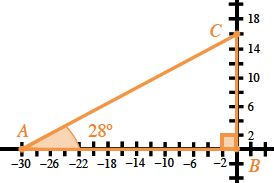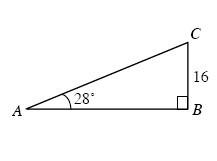### Home > A2C > Chapter 3 > Lesson 3.1.6 > Problem3-86

3-86.

Place the triangle at right on a set of $x$and $y$ axes so that $B$ is located at the origin.

1. Find the coordinates of $A$ and $C$.

Use the trigonometric ratios to find the other side lengths.2. Find the area and perimeter of triangle $ABC$.

Find the missing side lengths using the trigonometric ratios and then use those to find the area and perimeter.